Loading 2020 NBA Defensive Player of the Year Futures...
2020 NBA Defensive Player of the Year Odds
-335
0.3 to 1Rudy Gobert
54.0% implied probability

+230
2.3 to 1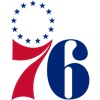Ben Simmons
21.3% implied probability

+700
7 to 1Myles Turner
8.8% implied probability

+3500
35 to 1Giannis Antetokounmpo
1.9% implied probability

+6000
60 to 1Joel Embiid
1.2% implied probability

+10000
100 to 1Draymond Green
0.7% implied probability

+10000
100 to 1Luguentz Dort
0.7% implied probability

+10000
100 to 1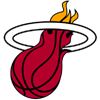Bam Adebayo
0.7% implied probability

+10000
100 to 1OG Anunoby
0.7% implied probability

+10000
100 to 1Fred VanVleet
0.7% implied probability

+10000
100 to 1Jrue Holiday
0.7% implied probability

+10000
100 to 1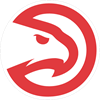Clint Capela
0.7% implied probability

+10000
100 to 1Paul George
0.7% implied probability

+10000
100 to 1Kawhi Leonard
0.7% implied probability

+15000
150 to 1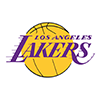LeBron James
0.5% implied probability

+15000
150 to 1Anthony Davis
0.5% implied probability

+15000
150 to 1Jimmy Butler
0.5% implied probability

+25000
250 to 1Deandre Ayton
0.3% implied probability

+25000
250 to 1Jayson Tatum
0.3% implied probability

+25000
250 to 1Jarrett Allen
0.3% implied probability

+25000
250 to 1Jaylen Brown
0.3% implied probability

+25000
250 to 1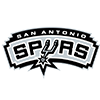Dejounte Murray
0.3% implied probability

+25000
250 to 1Patrick Beverley
0.3% implied probability

+50000
500 to 1Mitchell Robinson
0.1% implied probability

+50000
500 to 1Matisse Thybulle
0.1% implied probability

+50000
500 to 1Kevin Durant
0.1% implied probability

+50000
500 to 1Kyle Lowry
0.1% implied probability

+50000
500 to 1Marc Gasol
0.1% implied probability

+50000
500 to 1P.J. Tucker
0.1% implied probability

+50000
500 to 1Brook Lopez
0.1% implied probability

+50000
500 to 1Pascal Siakam
0.1% implied probability

+50000
500 to 1Donte DiVincenzo
0.1% implied probability

+50000
500 to 1Kyle Kuzma
0.1% implied probability

+50000
500 to 1DeAndre Jordan
0.1% implied probability

+50000
500 to 1Josh Richardson
0.1% implied probability

+50000
500 to 1Cameron Payne
0.1% implied probability

+50000
500 to 1Serge Ibaka
0.1% implied probability

+50000
500 to 1Kristaps Porzingis
0.1% implied probability

+50000
500 to 1Danny Green
0.1% implied probability

+50000
500 to 1Marcus Smart
0.1% implied probability

+50000
500 to 1Chris Paul
0.1% implied probability

+50000
500 to 1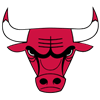Daniel Theis
0.1% implied probability

+50000
500 to 1Robert Covington
0.1% implied probability

+50000
500 to 1Steven Adams
0.1% implied probability

+50000
500 to 1Eric Bledsoe
0.1% implied probability

+50000
500 to 1Victor Oladipo
0.1% implied probability

+50000
500 to 1Khris Middleton
0.1% implied probability

+50000
500 to 1Jakob Poeltl
0.1% implied probability